# 2nd PUC Maths Previous Year Question Paper June 2018

Students can Download 2nd PUC Maths Previous Year Question Paper June 2018, Karnataka 2nd PUC Maths Model Question Papers with Answers helps you to revise the complete Karnataka State Board Syllabus and score more marks in your examinations.

## Karnataka 2nd PUC Maths Previous Year Question Paper June 2018

Time: 3 Hrs 15 Min
Max. Marks: 100

Instructions

• The question paper has five parts namely A, B, C, D, and E. Answer all the parts.
• Use the graph sheet for the question on Linear programming in Part – E

Part – A

Answer ALL the following questions: (10 × 1 = 10)

Question 1.
The relation R on set A = {1, 2, 3} is defined as R {(1, 1), (2, 2), (3, 3), (1, 2), (2, 3)} is not transitive. Why?
Solution:
(1, 2) ∈ R, (2, 3) ∈ R. But (1, 3) ∉ R

Question 2.
Write the range of y = cos-1 x
Solution:
[0, π]

Question 3.
If a matrix has 5 elements, what are the possible orders it can have?
Solution:
1 × 5, 5 × 1Question 4.
Find the values of x for which $$\left|\begin{array}{cc}x & 2 \\18 & x\end{array}\right|=\left|\begin{array}{cc}6 & 2 \\18 & 6\end{array}\right|$$
Solution:
x2 = 36 ⇒ x = ±6

Question 5.
Find $$\frac{d y}{d x}$$, if y = sin(ax + b)
Solution:
$$\frac{d y}{d x}$$ = a cos(ax + b)

Question 6.
Evaluate: ∫sec x (sec x + tan x) dx
Solution:
I = ∫sec2 x dx + ∫ sec x tan x dx = tan x + sec x + c

Question 7.
Define the negative of a vector.
Solution:
Negative of the vector is obtained by changing the direction of the given vector into the opposite direction or by multiplying the given vector by -1.
Say $$\vec{a}$$ be the given vector so negative of $$\vec{a}$$ is –$$\vec{a}$$
$$\vec{a}$$ and –$$\vec{a}$$ will have the same magnitude but opposite direction.

Question 8.
The Cartesian equation of a line is $$\frac{x-5}{3}=\frac{y-4}{7}=\frac{z-6}{2}$$. Write its vector form.
Solution:Question 9.
Define optimal solution in a linear programming problem.
Solution:
Any feasible solution of LPP which maximizes or minimizes the objective function is called an optimal solution.Question 10.
Find P(A/B), if P(B) = 0.5 and P(A∩B) = 0.32.
Solution: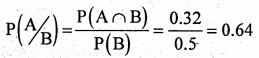Part – B

Answer any TEN questions: (10 × 2 = 20)

Question 11.
Define binary operation on a set. Verify whether the operation * is defined on Q set of rational numbers by a * b = ab + 1, ∀ a, b ∈ Q is binary or not.
Solution:
A binary operation on a set A is a function
* : A × A → A
a * b = ab + 1 ∈ Q
∴ * is a Binary Operation on Q.

Question 12.
Write $$\tan ^{-1}(\sqrt{\frac{1-\cos x}{1+\cos x}})$$, 0 < x < π in the simplest form.
Solution: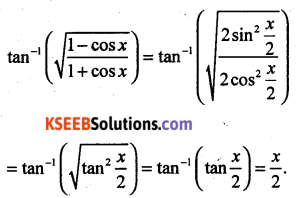Question 13.
Find the value of $$\cos ^{-1}\left(\cos \frac{13 \pi}{6}\right)$$
Solution: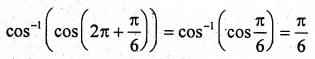Question 14.
If the area of the triangle with vertices (2, -6), (5, 4) and (K, 4) is 35 sq. units, then find the values of K, using determinants.
Solution: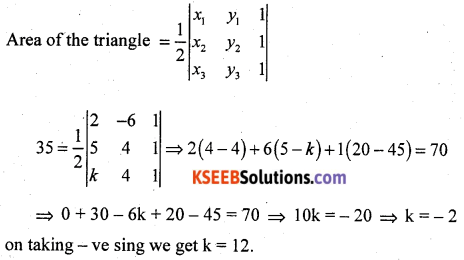Question 15.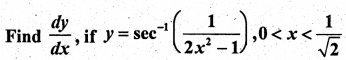Solution: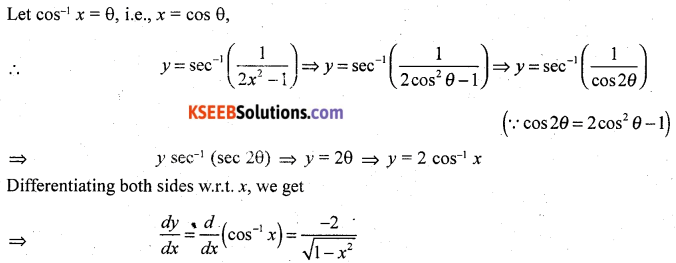Question 16.
Differentiate (sin x)cos x with respect to x.
Solution:Question 17.
If the radius of a sphere is measured as 7 m with an error of 0.02 m, then find the approximate error in calculating its volume.
Solution:
Let r be the radius of the sphere and ∆r be the error in measuring radius.
Then, r = 7 m and ∆r = 0.02 m
Now, volume of a sphere is given by V = $$\frac{4}{3} \pi r^{3}$$
On differentiate w.r.t r, we get $$\frac{d V}{d r}=\left(\frac{4}{3} \pi\right)\left(3 r^{2}\right)=4 \pi r^{2}$$
ΔV = ($$\frac{d V}{d r}$$) Δr
= (4πr2) Δr
= 4π × 72 × 0.002
= 3.92π m3
Hence, the approximate error in calculating the volume is 3.92π m3.Question 18.
Evaluate: $$\int \cos 6 x \sqrt{1+\sin 6 x} d x$$
Solution:Question 19.
Integrate $$\frac{x e^{x}}{(1+x)^{2}}$$ with respect to x.
Solution: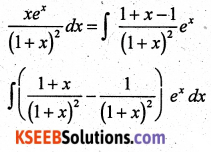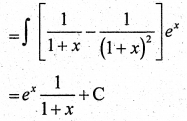Question 20.
Find the order and degree, if defined, of the differential equation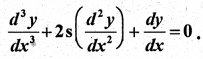Solution:
Order = 3, Degree = 1

Question 21.
Find the projection of the vector $$\vec{a}=\hat{i}-\hat{j}+3 \hat{k}$$ on the vector $$\vec{b}=2 \hat{i}+3 \hat{j}+2 \hat{k}$$.
Solution:Question 22.
Find the area of the parallelogram whose adjacent sides are given by the vectors $$\vec{a}=\hat{i}-\hat{j}+3 \hat{k}$$ and $$\vec{b}=2 \hat{i}-7 \hat{j}+\hat{k}$$
Solution: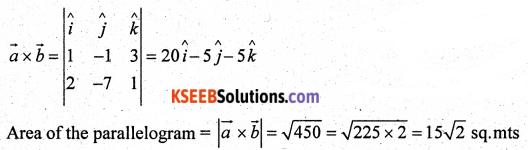Question 23.
Find the angle between the line $$\frac{x+1}{2}=\frac{y}{3}=\frac{z-3}{6}$$ and the plane 10x + 2y – 11z = 3
Solution: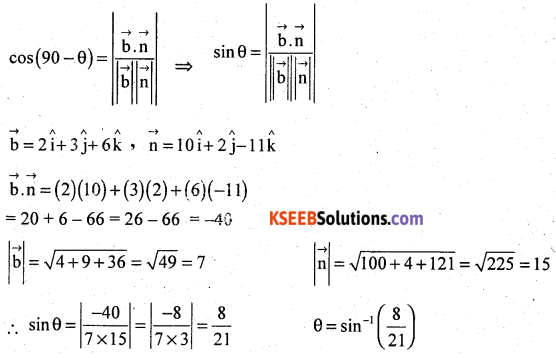Question 24.
The random variable X has a probability distribution P(X) of the following form, where K is some number.(a) Determine the value of K.
(b) Find P(X < 2).
Solution:
(a) ΣP(X) = 1
⇒ 6K = 1
⇒ K = $$\frac{1}{6}$$
(b) P(X < 2) = P(X = 0) + P(X = 1)
= K + 2K
= 3K
= 3($$\frac{1}{6}$$)
= $$\frac{1}{2}$$

Part – C

Answer any TEN questions: (10 × 3 = 30)

Question 25.
If f : R → R and g : R → R are given by f(x) = cos x and g(x) = 3x2, then show that gof ≠ fog.

Question 26.
Solve : tan-1 2x + tan-1 3x = $$\frac{\pi}{4}$$
Solution: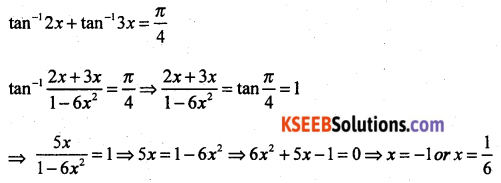Since x = -1 does not satisfy the equation, x = $$\frac{1}{6}$$ is the only solution of the given equation.

Question 27.
By using elementary operations, find the inverse of the matrix A = $$\left[\begin{array}{cc}3 & -1 \\-4 & 2\end{array}\right]$$
Solution:
A = I AQuestion 28.
If x = a(θ – sin θ) and y = a(1 + cos θ), then prove that $$\frac{d y}{d x}=-\cot (\theta / 2)$$
Solution:
Given x = a(θ – sin θ) and y = a(1 + cos θ)
Differentiating w.r.t θ, we get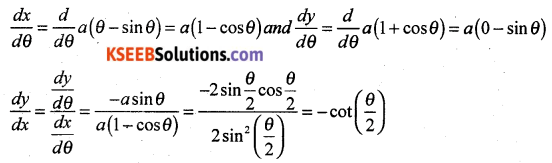Question 29.
Verify Mean Value Theorem if f(x) = x2 – 4x + 3 in the interval x ∈ [a, b], where a = 1 and b = 4.
Solution:
f(x) is a polynomial in x.
It is continuous in {1, 4} and differentiable in {1, 4) and f'(x) = 2x – 4.
There exists at least one value c ∈ (1, 4) such that f'(c) = $$\frac{f(b)-f(a)}{b-a}$$
a = 1, f(a) = f(1) = 12 – 4(1) – 3 = 1 – 4 – 3 = 1 – 7 = -6
b = 4, f(b) = f(4) = 42 – 4(4) – 3 = 16 – 16 – 3 = -3
f'(c) = 2c – 4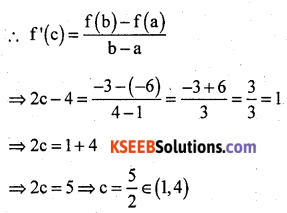∴ Mean Value theorem is verified.Question 30.
Find two positive numbers whose sum is 15 and the sum of whose squares is minimum.

Question 31.
Evaluate: $$\int_{0}^{1} \frac{\tan ^{-1} x}{1+x^{2}} d x$$
Solution:Question 32.
Integrate $$\frac{d x}{x\left(x^{2}+1\right)}$$ with respect to x.
Solution:Question 33.
Find the area of the parabola y2 = 4ax bounded by its latus rectum.
Solution:Question 34.
Find the differential equation representing the family of curves y = a sin(x + b), where a, b are arbitrary constants.Question 35.
Find a unit vector perpendicular to each of the vectors $$(\vec{a}+\vec{b})$$ and $$(\vec{a}-\vec{b})$$ where $$\vec{a}=3 \hat{i}+2 \hat{j}+2 \hat{k}$$ and $$\vec{b}=\hat{i}+2 \hat{j}-2 \hat{k}$$
Solution: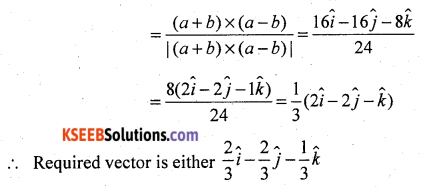Question 36.
Prove that $$[\vec{a}+\vec{b}, \vec{b}+\vec{c}, \vec{c}+\vec{a}]=2[\vec{a}, \vec{b}, \vec{c}]$$
Solution: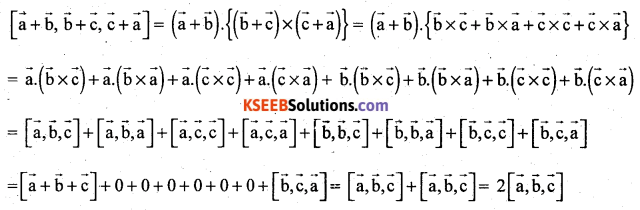Question 37.
Find the equation of the plane through the intersection of the planes 3x – y + 2z = 0 and x + y + z – 2 = 0 and the point (2, 2, 1)

Question 38.
A man is known to speak truth 4 out of 5 times. He tossed a coin and reports that it is head. Find the probability that it is actually head.
Solution:
E1 : coin shows a head
E2 : coins shows a tail
P(E1) = P(E2) = $$\frac{1}{2}$$
S = {H, T}
E1 = {H}, E2 = {T}
Let E : A reports that a head appears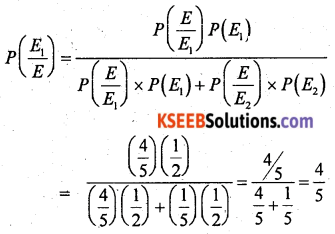Part – D

Answer any SIX questions: (6 × 5 = 30)

Question 39.
Let R+ be the set of all non-negative real numbers. Show that the function f : R+ → [4, ∞] given by f(x) = x2 + 4 is invertible and write the inverse of f.
Solution:Question 40.
If A = $$\left[\begin{array}{c}1 \\-4 \\3\end{array}\right]$$, B = $$\left[\begin{array}{lll}-1 & 2 & 1\end{array}\right]$$, verify that (AB)’ = B’A’. Calculate AC, BC and (A + B)C. Also, verify that (A + B)C = AC + BC.
Solution: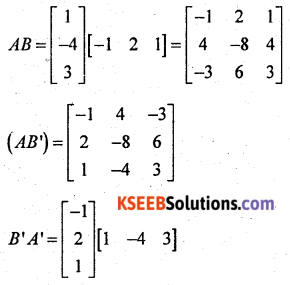Question 41.
Solve the following system of equations by matrix method.
4x + 3y + 2z = 60
2x + 4y + 6z = 90
6x + 2y + 3z = 70
Solution:
This system of equations can be written as AX = B, where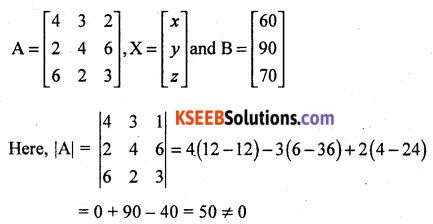Thus, A is non-singular, Therefore, its inverse exists.
Therefore, the given system is consistent and has a unique solution given by X = A-1 B.
Cofactors of A are
A11 = 12 – 12 = 0
A12 = -(6 – 36) = 30
A13 = 4 – 24 = -20
A21 = -(9 – 4) = -5
A22 = 12 – 12 = 0
A23 = -(8 – 18) = 10
A31 = (18 – 8) = 10
A32 = -(24 – 4) = -20
A33 = 16 – 6 = 10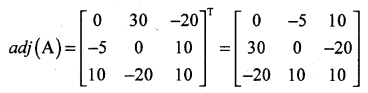Question 42.
If Y = A emx + B enx show that $$\frac{d^{2} y}{d x^{2}}-(m+n) \frac{d y}{d x}+m n y=0$$
Solution: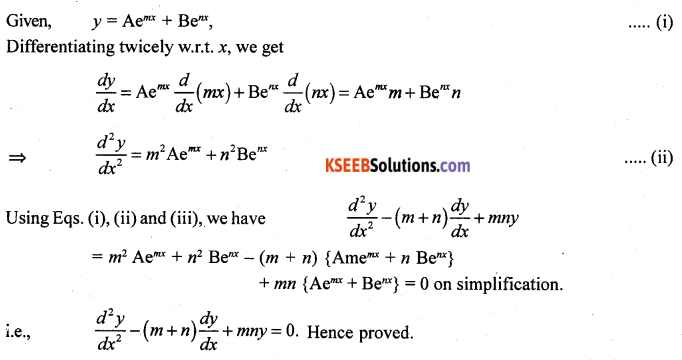Question 43.
A particle moves along the curve 6y = x3 + 2. Find the points on the curve at which y-coordinate is changing 8 times as fast as the x-coordinate.
Solution: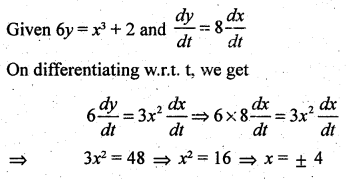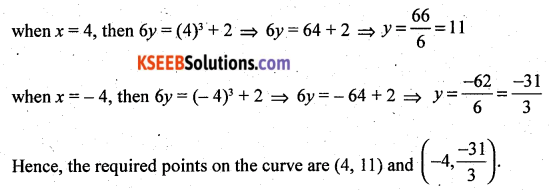Question 44.
Find the integral of $$\frac{1}{\sqrt{a^{2}-x^{2}}}$$ with respect to x and hence find $$\int \frac{1}{\sqrt{7-6 x-x^{2}}} d x$$
Solution: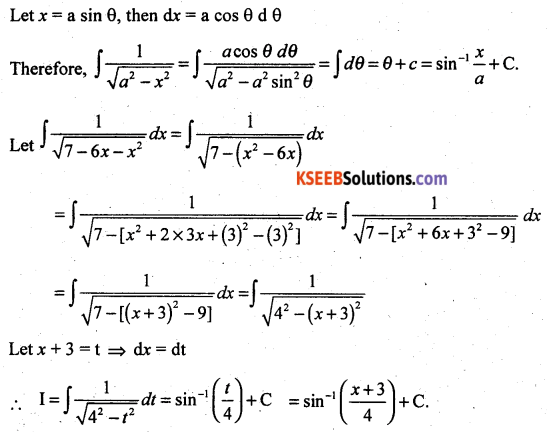Question 45.
Find the area eiiclosed by the ellipse $$\frac{x^{2}}{a^{2}}+\frac{y^{2}}{b^{2}}=1$$ by the method of integration.
Solution: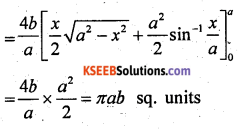Question 46.
Find the general solution of the differential equation x $$\frac{d y}{d x}$$ + 2y = x2, (x ≠ 0)
Solution:Question 47.
Derive the equation of a line in space passing through two given points both in vector and Cartesian form.
Solution:
Vector Form
Let $$\overrightarrow{\mathrm{a}}$$ and $$\overrightarrow{\mathrm{b}}$$ be the position vectors of two points A(x1, y1, z1) and B(x2, y2, z2) respectively that are lying on a line.
Let $$\overrightarrow{\mathrm{r}}$$ be the position vector of an arbitrary point P (x, y, z), then P is a point on the line if and only if $$\overrightarrow{\mathrm{AP}}=\overrightarrow{\mathrm{r}}-\overrightarrow{\mathrm{a}}$$ and $$\overrightarrow{\mathrm{AB}}=\overrightarrow{\mathrm{b}}-\overrightarrow{\mathrm{a}}$$ are collinear vectors. Therefore, P is on the line if an only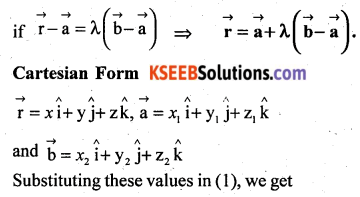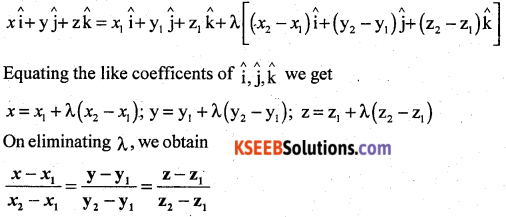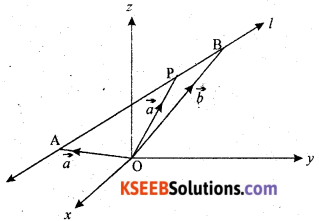Question 48.
A person buys a lottery ticket in 50 lotteries, in each of which his chance of winning a prize is $$\frac{1}{100}$$. What is the probability that he will win a prize
(a) at least once
(b) exactly once
Solution:
Let X denote the number of winsPart – E

Answer any ONE question: (1 × 10 = 10)

Question 49(a).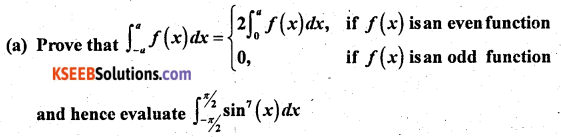Solution: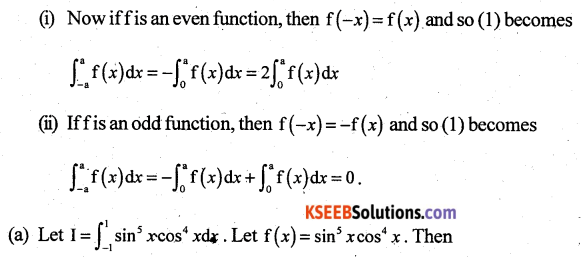Question 49(b).
Find the value of K, ifSolution:Question 50(a).
Solve the following problem graphically:
Maximize and minimize
Z = 10500x + 9000y
Subject to the constraints
x + y ≤ 50
2x + y ≤ 80
x ≥ 0, y ≥ 0
Solution:
x + y = 50
y = 0 ⇒ x = 50
∴ A = (50, 0), B = (0, 50)
(0, 0) lies on x + y ≤ 50
2x + y = 80
y = 0, x = 40 ⇒ C(40, 0)
x = 0, y = 80 ⇒ D(0, 80)
Origin lies on 2x + y ≤ 80
Solving x + y = 50
2x + y = 80 we get x = 30, y = 20
E = (30, 20)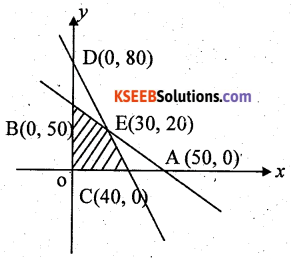OBEC is the feasible region.Question 50(b).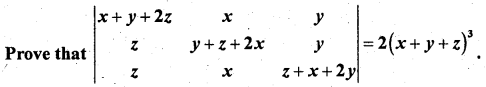Solution: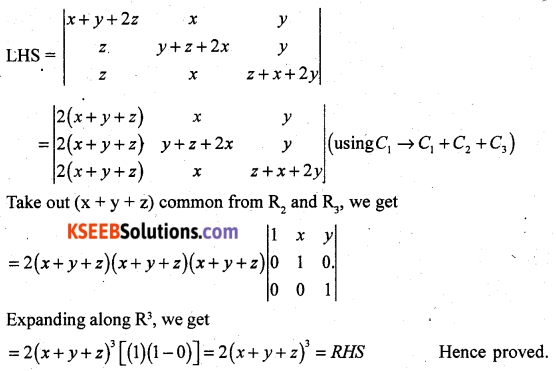error: Content is protected !!